Courses

# Motion of a Particle in a Central Potential - General Formalism of Wave Mechanics, Quantum Mechanics Physics Notes | EduRev

## Physics for IIT JAM, UGC - NET, CSIR NET

Created by: Akhilesh Thakur

## Physics : Motion of a Particle in a Central Potential - General Formalism of Wave Mechanics, Quantum Mechanics Physics Notes | EduRev

The document Motion of a Particle in a Central Potential - General Formalism of Wave Mechanics, Quantum Mechanics Physics Notes | EduRev is a part of the Physics Course Physics for IIT JAM, UGC - NET, CSIR NET.
All you need of Physics at this link: Physics

Consider a spinless particle of mass m in a central potential V(r).  The potential energy of the particle depends only on its distance from the origin.

For a classical particle in a central potential the force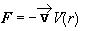is always directed towards the origin, the torque t=r´F  is zero, and the angular momentum L=r´p is a constant of motion.  The particle's trajectory lies in a plane passing through the origin.  Its velocity v may be decomposed into a component vr along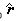and a component v^ perpendicular toin this plane.  The energy of the particle is given by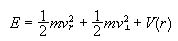.

Using p=mv and L=mrv^ we can write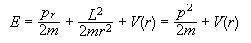with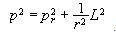.

To make the transition to quantum mechanics we write down the quantum-mechanical Hamiltonian H of the particle by replacing in the expression for E the classical physical quantities expressed in terms of r and p by their corresponding quantum mechanical operators, symmetrizing suitably, if necessary.  In coordinate representations we have already found expressions for the operators px, py, pz, p2, and L2, but we have not yet found an expression for pr2.

In coordinate representation we write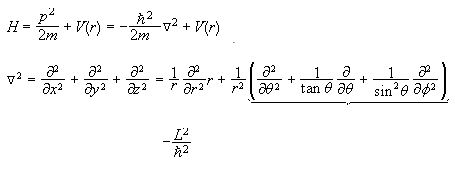.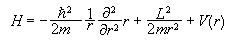The expression for pr2 in coordinate representation therefore is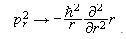.

To find the wave functions y(r,q,f) of the eigenstates of H we have to solve the eigenvalue equation Hy(r)=Ey(r).  For a particle in a central potential the entire angular dependence of H is contained in the L2 term.  L2 commutes with all components Li of L.  All other terms of H depend only on r, not on q and f, and commute with Li.  We have [H,Li]=0, [H,L2]=0.  The angular momentum L of the particle is therefore a constant of motion.  We can find a common eigenbasis of H, L2 and Lz.  We denote the states of this basis by |k,l,m> and the corresponding eigenfunctions by yklm(r,q,f).  We have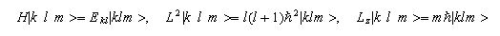.

We already know the general form of yklm(r,q,f).  yklm(r,q,f)=Rkl(r)Ylm(q,f) is a product of a radial function Rkl(r) and the spherical harmonic Ylm(q,f).  (See notes!)

Substituting this form of yklm(r,q,f) into the eigenvalue equation yields the differential equation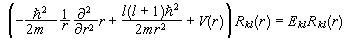for Rkl(r).

Note: Since H commutes with L±E cannot depend on m.  E is therefore at least (2l+1) fold degenerate.  Accidental degeneracies may add to this essential degeneracy.

Writing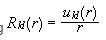we find a differential equation for ukl(r), which has a simpler form than the differential equation for Rkl(r).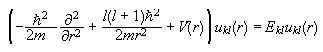.

This differential equation has the same form as the one-dimensional Schroedinger equation for a particle moving in an effective potential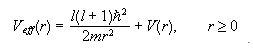.

(Here is one reason why we have spend so much time on 1-d problems.)

But r is not x.  The variable r can take on only non-negative real values.  R(r) has to stay finite at r=0, therefore u(r) has to go to zero at r=0.  Otherwise y(r,q,f) is not an acceptable wave function.  Choosing Veff(r)=¥ for r<0 lets us treat the radial equation as an ordinary one-dimensional equation while guaranteeing the proper behavior at the origin.

#### The asymptotic behavior of Rkl(r)

Assume Rkl(r) is sufficiently regular that it may be expanded in powers of r near the origin.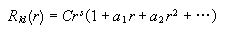,

where s is the lowest power with a non-zero coefficient.

Therefore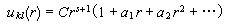.

Substituting this expression for ukl(r) into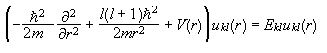yields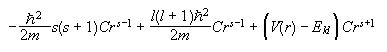+ (terms containing powers of r greater than s-1) = 0, if |V(r)|£ C'r-2 as r®0.

The coefficients of successive powers of r have to vanish independently, since this equation holds for all r.  Letting the coefficient of rs-1 vanish we obtain -s(s+1)+l(l+1)=0 if |V(r)|< C'r-2 as r®0, i.e. if |V(r)| does not blow up as fast as r-2 as r®0.  This is a quadratic equation with two solutions.  It implies that s=l, or that s=-(l+1) if |V(r)|< r-2 as r®0.  As r®0 the lowest power of r dominates and ukl(r)®Crl+1.  The solution ukl(r)®Crl+1 goes to zero at r=0, but the solution ukl(r)®Cr-l blows up at r=0 and is unacceptable.

Near the origin the radial behavior of an acceptable wave function of a particle moving in a central potential is proportional to rl, (if |V(r)|<C'r-2). Note: R(r)=u(r)/r.

#### Two interacting particles

Consider an isolated system of two interacting spinless particles of masses m1 and m2 at positions r1 and r2, respectively.  The potential energy of the particles depends only on their relative position r1-r2.  In classical mechanics the Lagrangian of such a particle can be written as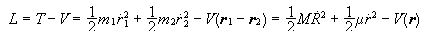,

where M=m1+m2 is the total mass of the system,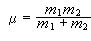is the reduced mass of the system,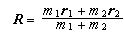are the coordinates of the center of mass of the system,

and r=r1-r2 are the relative coordinates.

Using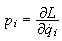we define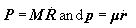.  The classical equations of motion are obtained from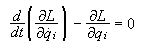.

They are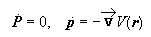.

If we take PR and pr as the new canonical variables of the system then the Hamiltonian of the system is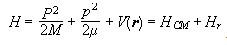.

The first term represents the kinetic energy associated with the motion of the center of mass, which is in uniform rectilinear motion ( P = 0).  The other terms represent the energy associated with the relative motion.  If we choose an inertial frame in which the center of mass is at rest, then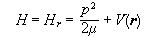is the Hamiltonian of a fictitious particle with mass m, whose position is given by the relative coordinate r, moving in an external potential V(r).  The relative motion of two interacting spinless particles reduces to the motion of a single fictitious particle in an external potential.

To make the transition to quantum mechanics we again replace in the expression for H the classical physical quantities expressed in terms of the canonical variables by their corresponding quantum mechanical operators and symmetrize suitably, if necessary.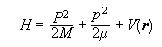,

where H, Pp, and r are now operators.  Expressing these operators in terms of p1p2r1, and r2 and using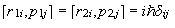we can show that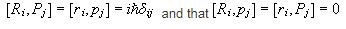for all i and j.

We therefore have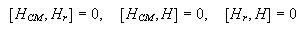.

There exists a common eigenbasis of H, HCM, and Hr.  We may consider the state space E to be the tensor product of ECM and Er, E=ECMÄEr.  P and R operate only in ECM and p and r operate only in Er.  The motion of the center of mass and the relative motion are completely independent of each other.

Let {|c>} be an eigenbasis of HCM and {|w>} be an eigenbasis of Hr.  Then {|f>=|c>Ä|w>}is an eigenbasis of H in E.

,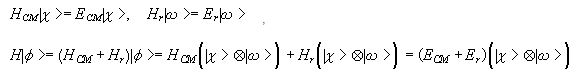.

In coordinate representation we write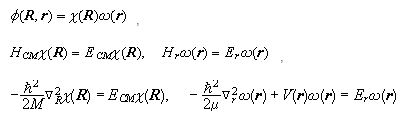We can solve for c(R).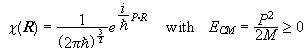.

The fictitious particle associated with the motion of the center of mass behaves like a free particle and is represented by a plane wave.

We are often only interested in the relative motion, i.e. in the behavior of the two interacting particles in their center of mass frame.  If their mutual interaction depends only on the distance between them, then V(r)=V(r) and the eigenvalue equation becomes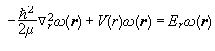.

Then

.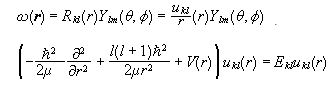.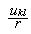is the radial function of a particle of mass m moving in a central potential.

#### Problem:

Assume that the potential energy of the deuteron is given by V(r)=-V0, r<r0; V(r)=0 r³r0.

(a) Show that the ground state of the deuteron possesses zero orbital angular momentum (l=0).  Since this is true for any central potential, you may not need the detailed nature of the square well potential.

(b) Assume that l=0 and estimate the value of V0 under the additional condition that the value of the binding energy is much smaller than V0.

Solution:

(a)  The Hamiltonian of the relative motion of the two particles is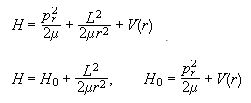Let yl be the lowest energy eigenfunction of H with orbital angular momentum quantum number l.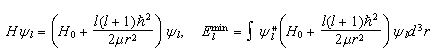.

Let yl+1 be the lowest energy eigenfunction of H with orbital angular momentum quantum number l+1.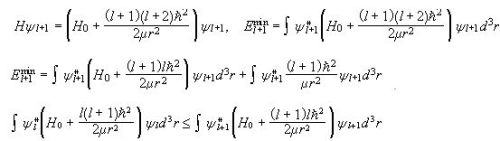since yl is assumed to be the lowest energy eigenfunction of the Hamiltonian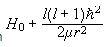.

Therefore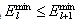and the ground state possesses zero orbital angular momentum.

(b)  The relative motion of the two particles is described in the same way as the motion of a fictitious particle of reduced mass m in a central potential.  Therefore we have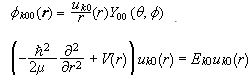.

The problem is reduced to a one dimensional "square well problem" with E<0.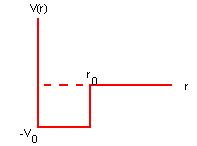Let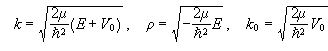.

Let r<r0 define region 1 and r>r0 define region 2.  The coordinate r is never negative.  Therefore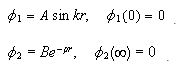.

The boundary conditions are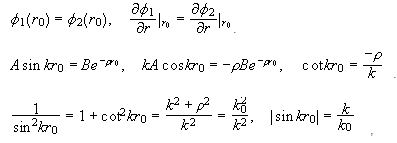in regions where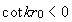.

If we plot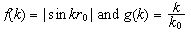we find solutions at the intersections of the two curves in regions where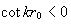, i.e.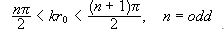.

For only one solution to exist we need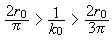.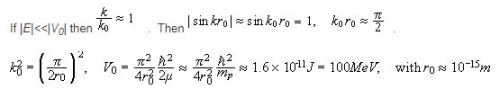(Rule of thumb: To estimate the range of a force, divide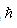by the mass of the particle that carries it times c,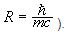).

159 docs

,

,

,

,

,

,

,

,

,

,

,

,

,

,

,

,

,

,

,

,

,

,

,

,

;